## BIG TIPPER [DAY 2]

### PERCENT AND FINANCIAL LITERACY

Investigate strategies to increase and decrease a whole number by a percent.

## Intentionality & Unit Overview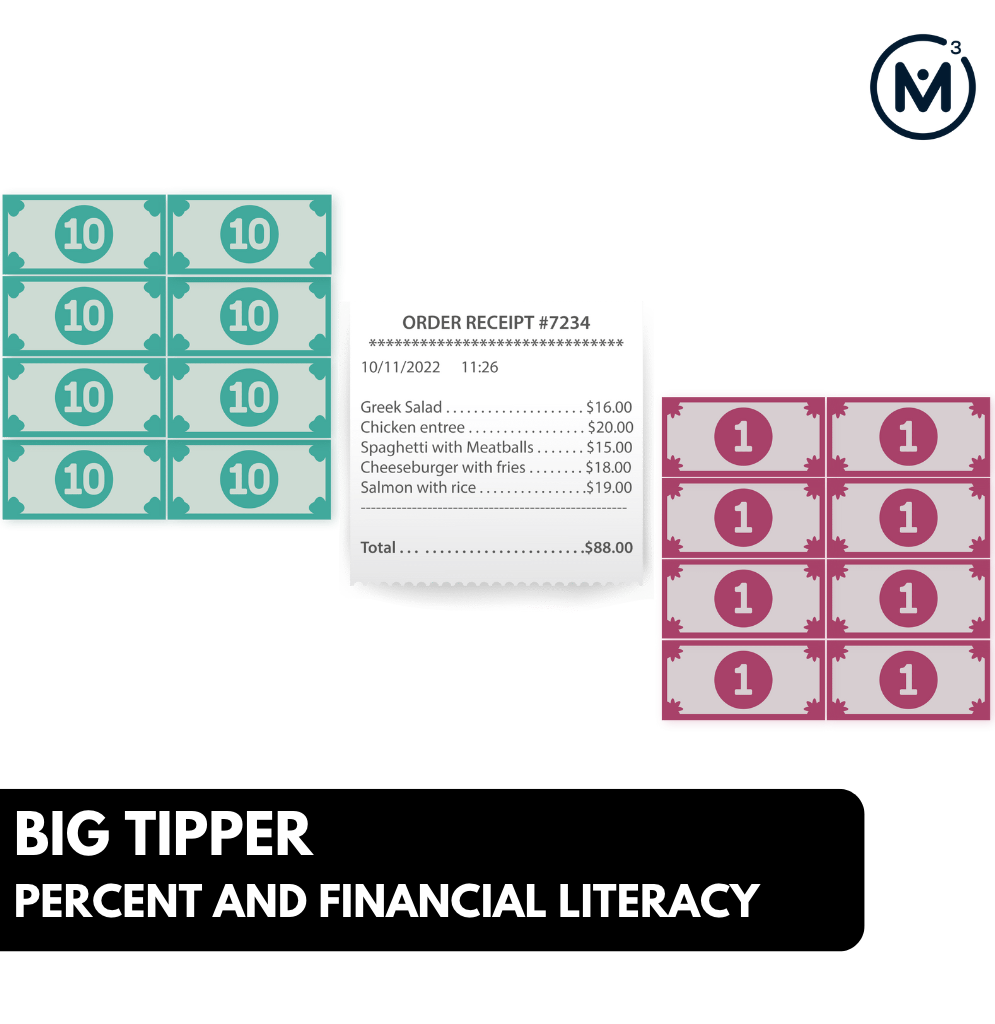### Access each lesson from this unit using the navigation links below

Students will continue exploring percent as operator in topics related to financial literacy. Students will use mental math strategies to increase a whole number by a percent. They will need to understand and recall commonly used percent and fraction equivalents.

## Intentionality…

The purpose of the Day 2 activities is to reinforce key concepts from Day 1. Students will engage in a math talk and will have an opportunity to complete independent purposeful practice. The math talk and purposeful practice serve to develop a deeper understanding of the following big ideas.

•  Fractions, decimals, and percents can all represent relationships to a whole;
• Certain equivalent representations of percents and fractions are more helpful to do mental calculations than others;
• Any fraction, decimal or percent can be used as an operator;
• Increasing by a given percent means the percent is added to the whole;
• A bar model is helpful for representing a situation that involves a percent increase.

## Math Talk

The purpose of today’s visual number talk is to understand and recall commonly used percent and fraction equivalents. Percents such as 1%, 10%, 25%, 50% and 100% are helpful when using mental math strategies to increase a whole number by a percent. In today’s math talk, students will draw conclusions about an individual’s monthly spending habits by interpreting the information in a stacked bar graph and a circle graph. As students are sharing their conclusions, for example, “this individual spends half of their income on housing”, encourage students to communicate this conclusion as a fraction and as a percent.Leverage the stacked bar graph/circle graph to justify the equivalence.

## Visual Math Talk Prompt #1

Begin by showing the following silent animation video:

After the initial context is set up, be prepared to pause the video where students are prompted with a question to struggle with.

Prompt:

This stacked bar graph represents an individual’s monthly spending. What conclusions can be made about the individual’s spending? Use fractions and percents to communicate your conclusions.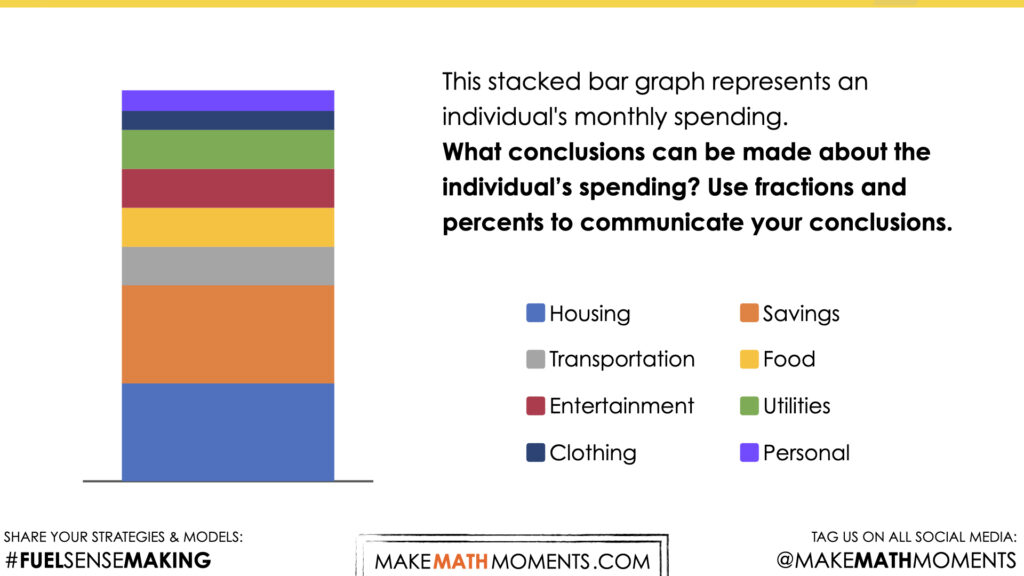Continue the video and ask the student’s to refine their thinking now that the bar graph shows the partitions.

Were their estimates correct?

Can they be more definitive with their conclusions?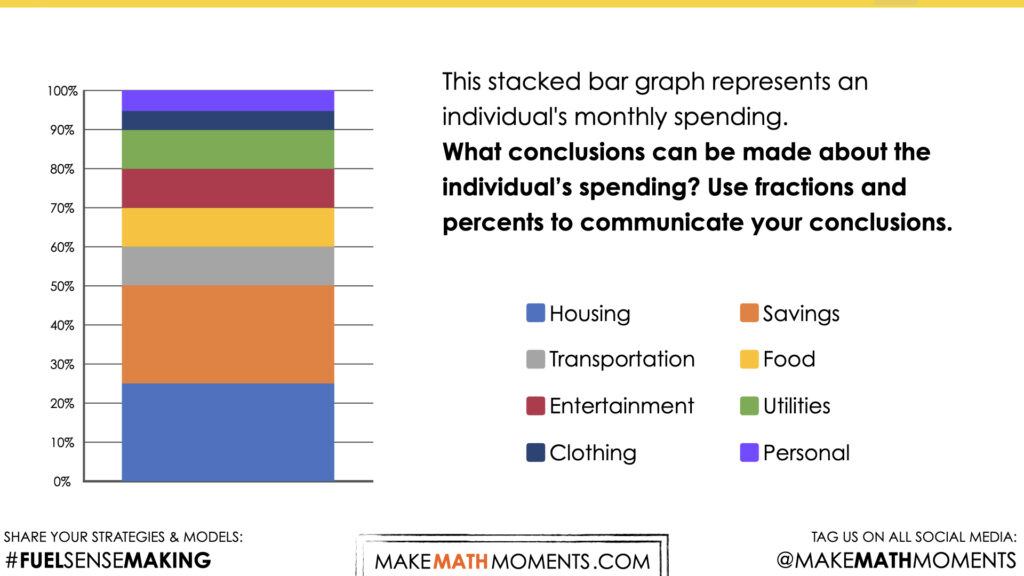## Visual Math Talk Prompt #2

Begin by showing the following silent animation.

Pause the video where students are prompted with a question to struggle with.Then ask students:

Prompt:

This circle graph represents another individual’s monthly spending. What conclusions can be made about the individual’s spending? Use fractions and percents to communicate your conclusions.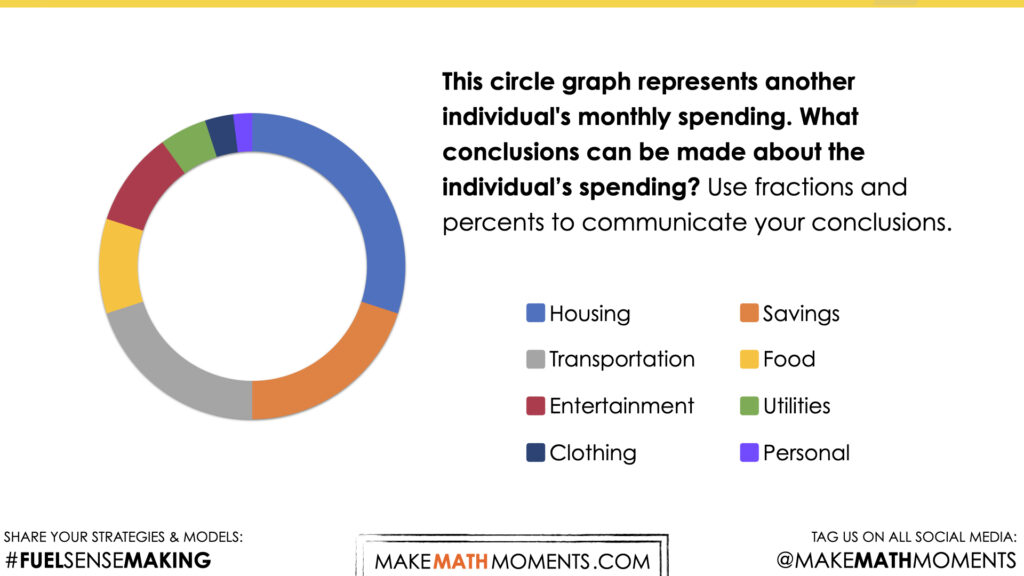Continue the video and ask the student’s to refine their thinking now that the circle graph has been stretched into a horizontal stacked bar graph.

Were their estimates correct?

Can they be more definitive with their conclusions?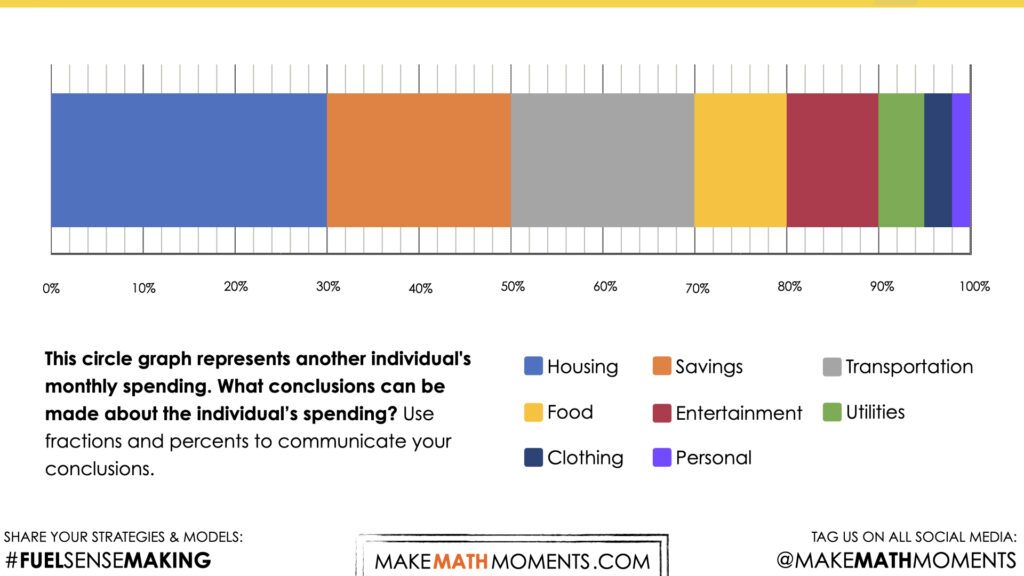## Purposeful Practice

Login/Join to access the entire Teacher Guide, downloadable slide decks and printable handouts for this lesson and all problem based units.

## Questions: Increasing a Whole Number by a Percent

Login/Join to access the entire Teacher Guide, downloadable slide decks and printable handouts for this lesson and all problem based units.

Login/Join to access the entire Teacher Guide, downloadable slide decks and printable handouts for this lesson and all problem based units.

## Educator Discussion Area

Login/Join to access the entire Teacher Guide, downloadable slide decks and printable handouts for this lesson and all problem based units.

## Explore Our 60+ Problem Based Units

This Make Math Moments Lesson was designed to spark curiosity for a multi-day unit of study with built in purposeful practice, number talks and extensions to elicit and emerge strategies and mathematical models.

Dig into our other units of study and view by concept continuum, grade or topic!